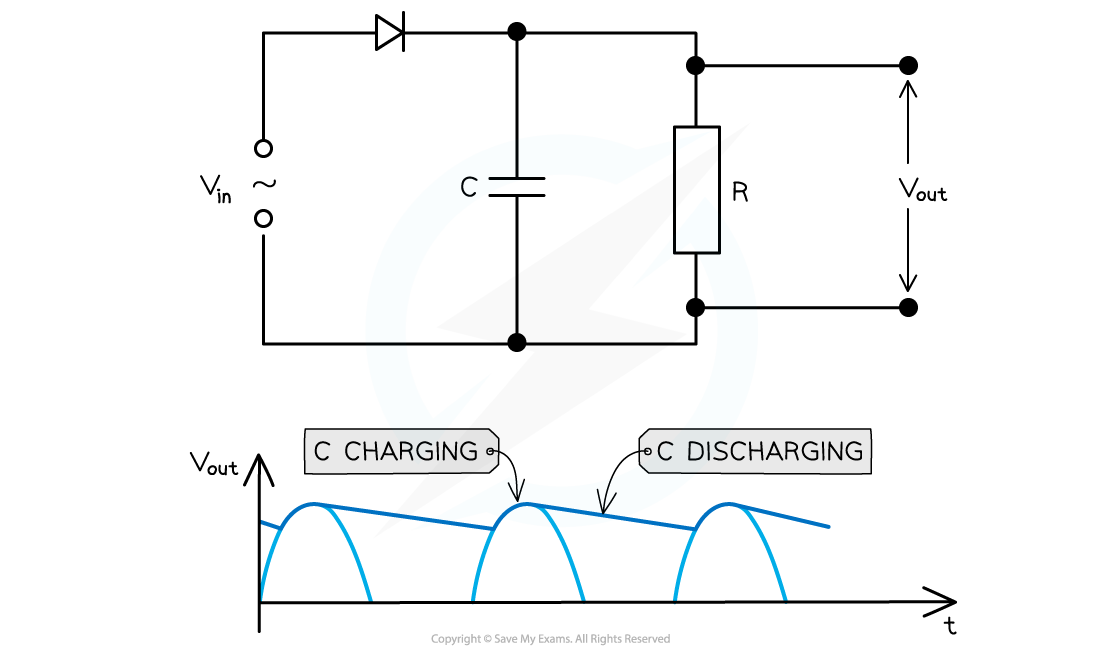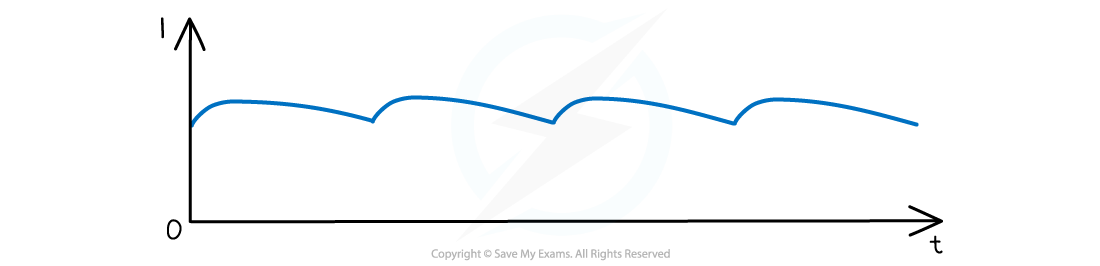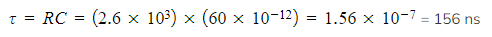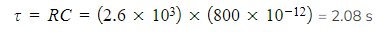# IB DP Physics: HL复习笔记11.2.9 Capacitors & Diode Bridges

### Capacitors & Diode Bridges

• In rectification, to produce a steady direct current  or voltage from an alternating current  or voltage, a smoothing capacitor is necessary
• Smoothing is defined as:

The reduction in the variation of the output voltage or current

• This works in the following ways:
• A single capacitor with capacitance C is connected in parallel with a load resistor of resistance R
• The capacitor charges up from the input voltage and maintains the voltage at a high level
• It discharges gradually through the resistor when the rectified voltage drops but the voltage then rises again and the capacitor charges up againA smoothing capacitor connected in parallel with the load resistor. The capacitor charges as the output voltage increases and discharges as it decreases

• The resulting graph of a smoothed output voltage Vout and output current against time is a ‘ripple’ shapeA smooth, rectified current graph creates a ‘rippling’ shape against time

• The amount of smoothing is controlled by the capacitance C of the capacitor and the resistance R of the load resistor
• The smaller the rippling effect, the smoother the rectified current and voltage output
• The slower the capacitor discharges, the more the smoothing that occurs ie. smaller ripples
• This can be achieved by using:
• A capacitor with greater capacitance C
• A resistor with larger resistance R
• Recall that the product RC is the time constant τ of a resistor
• This means that the time constant of the capacitor must be greater than the time interval between the adjacent peaks of the output signal

#### Worked Example

The graph below shows the output voltage from a half-wave rectifier. The load resistor has a resistance of 2.6 kΩ. A student wishes to smooth the output voltage by placing a capacitor in parallel across the load resistorSuggest if a capacitor of 60 pF or 800 µF would be suitable for this task

Step 1:

Calculate the time constant with the 60 pF capacitorStep 2:

Compare time constant of 60 pF capacitor with interval between adjacent peaks of the output signal

• The time interval between adjacent peaks is 80 ms
• The time constant of 156 ns is too small and the 60 pF capacitor will discharge far too quickly
• There would be no smoothing of the output voltages
• Therefore, the 60 pF capacitor is not suitable

Step 3:

Calculate the time constant with the 800 µF capacitorStep 4:

Compare time constant of 800 pF capacitor with interval between adjacent peaks of the output signal

• The time constant of 2.08 s is much larger than 80 ms
• The capacitor will not discharge completely between the positive cycles of the half-wave rectified signal
• Therefore, the 800 µF capacitor would be suitable for the smoothing task